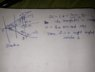# Triangle formed by tangents to a parabola

• takando12
In summary: Anyway, to answer your question, the triangle formed by the tangents to the parabola y2=4ax at the ends of the latus rectum and the double ordinate through its focus is always a right angled isosceles triangle. The key here is to remember that the slope of a tangent to a parabola at a given point is always equal to the reciprocal of the slope of the line joining the point to the focus. This means that the slope of the tangents at the points (a,2a) and (a,-2a) is -1/2a. The line joining these two points is the latus rectum, which has a slope of -1/a. Since the latus

## Homework Statement

The triangle formed by the tangents to the parabola y2=4ax, at the ends of the latus rectum and the double ordinate through it's focus is:
1) equilateral 2) acute angles isosceles 3)right angled isosceles 4) dependent on value of a

## The Attempt at a Solution

The double ordinate through the focus is the latus rectum. I tried to find the intersection of the two tangents by using the AM and GM of the co-ordinates of the points of contact with the parabola .
the two points of contact : (a,2a) and (a,-2a)
x-coordinate of intersection = GM=√a2=a
y-coordinate of intersection = AM= 2a-2a/2 =0
Which gives me the intersection of the two tangents to be (a,0) which is the co-ordinates of the focus. This is clearly wrong. Where am I going wrong in my approach?

Maybe you should post how you compute(d) the slope of the tangents at the two points of contact.

takando12 said:

## Homework Statement

The triangle formed by the tangents to the parabola y2=4ax, at the ends of the latus rectum and the double ordinate through it's focus is:
1) equilateral 2) acute angles isosceles 3)right angled isosceles 4) dependent on value of a

## The Attempt at a Solution

The double ordinate through the focus is the latus rectum. I tried to find the intersection of the two tangents by using the AM and GM of the co-ordinates of the points of contact with the parabola .
the two points of contact : (a,2a) and (a,-2a)
x-coordinate of intersection = GM=√a2=a
y-coordinate of intersection = AM= 2a-2a/2 =0
Which gives me the intersection of the two tangents to be (a,0) which is the co-ordinates of the focus. This is clearly wrong. Where am I going wrong in my approach?
Since x = √a2 Clearly a is not equal to +a therefore the the abcissa is -a.
And the option three is correct

#### Attachments

•15582726089115062018012970823886.jpg
59.3 KB · Views: 178
Have you noticed you are replying to a post that was made almost 3 years ago?